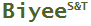Tweet

# Confidence Interval

## -widely used, rarely truly understood

(last updated on 2011-02-23)

Confidence interval is one of the most widely used statistic terms. Its use goes well beyond the research community. One ubiquitous term derived from confidence interval is margin of error which is the radius of a confidence interval. It is used in all kinds of polls from consumer opinions to political elections.

Unfortunately, users of confidence intervals rarely truly understand exactly what they mean though it does not mean they use them incorrectly.  Fortunately, some vague understanding of this statistic is sufficient for using it which is often a part of standard data analysis procedures in many fields.  Wikipedia opens the article on confidence interval by saying "In statistics, a confidence interval (CI) is a particular kind of interval estimate of a population parameter and is used to indicate the reliability of an estimate."  This general interpretation may be all one needs to know about the confidence interval. The mathematical definition of confidence interval as given by theWikipedia article is convoluted, but it is necessary to maintain the rigor; so it does not help most people further their understanding of this term. All kinds of discussions and debates on the interpretation of this term can be readily found online providing further evidence that this term has generated many confusions.

This article provides an easy and accurate interpretation of  the term confidence interval from a user's, not a mathematician's, point of view.

A confidence interval has two parts: the confidence level and the interval.  The interval is straightforward - a range of values. The confidence level is what from which all confusions come.  The confidence level is a probability, but this probability is not of the measure that the interval encompass. It is a probability about the method used to compute the interval.  Let us use an example to clarify all the terms.

The cholesterol level of the US population has been surveyed extensively. First, let us describe the data in statistical terms and the correct way. The average is about 215 mg/dL, and 95% of the data are between 150 and 280 mg/dL. Here, the number 95% is not a confidence level, and [150, 280] is not a confidence interval.

Now, let us assume that the population's cholesterol data is unknown and a survey has been completed to estimate the average cholesterol level.  The mean of the sample is 210, the standard deviation is 30, and the sample size is 100. Based on these data, using 90% as the confidence level, the confidence interval calculated to be [210, 220]. How should we interpret these data? One common mistake would be saying that the average cholesterol of the population has a 90% chance of being within the range of 210 to 220.  This is incorrect because the population average is a single number.  It has 100% chance of being a single value though we do not know exactly what it is. The correct interpretation is the following:

The population average is assessed to be a value between 210 and 220 by a method.  The method has a success rate of 90% in making such assessment.

In other words, if the survey is repeated many times, each will generate a different interval using the same method, and 90% of the intervals will capture the true average.  Of course, in reality, one would in this case aggregate all the data to generate a much narrower interval.

Related: